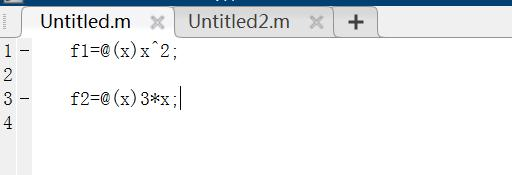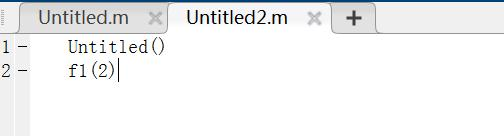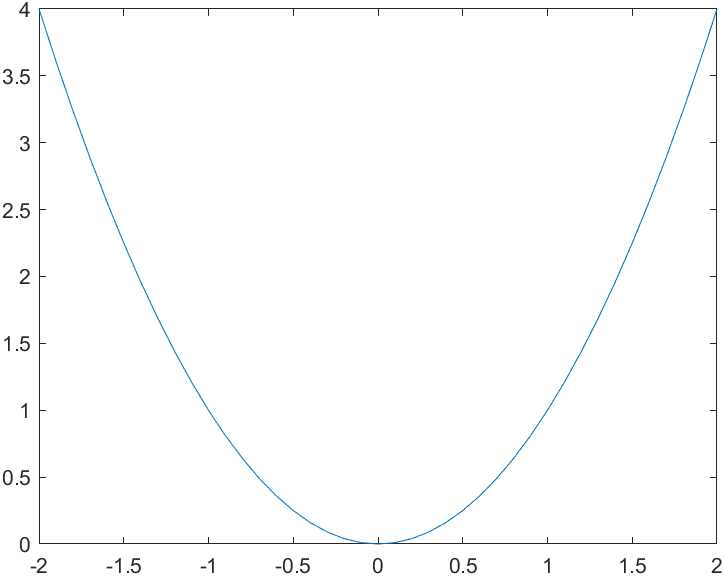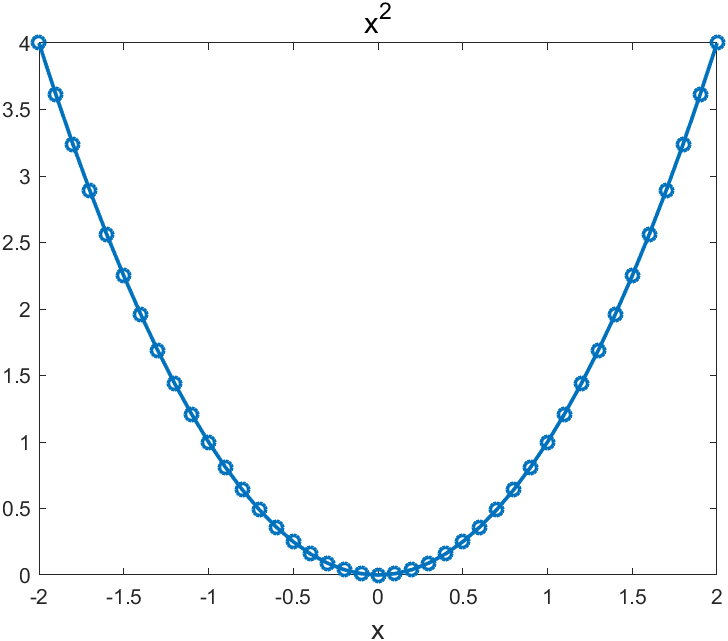• matlab匿名函数的用法
2022-05-07 18:24:53

matlab匿名函数的基本使用是

变量名=@（自变量）函数

一.如果函数是自己定义的函数 如x^2+2*x+1

若自变量是数

f=@(x) x^2+2*x+1

则f（1）=4

若自变量是向量

f=@(x) x.^2+2*x+1

则f（1：5）=4 9 16 25 36

注意向量之间的运算

二.如果是matlab内部的函数如sin(x)

可表示为

f=@(x) sin(x)

或者f=@ sin

则f(pi/2)=1

对于改例子自变量为数或者向量形式都可matlab
更多相关内容
• ## MATLAB匿名函数详解（超级全面）

万次阅读 多人点赞 2021-03-04 19:14:00
本篇文章主要讲解MATLAB 匿名函数的一些基础的及独特的用法： 目录1 匿名函数的生成1.1基础生成1.2 多参数匿名函数1.3 含定参匿名函数1.4 sym转匿名函数1.5 字符串转匿名函数1.6 函数工厂（匿名函数的自动生成）...

匿名函数与之相对的即为需要.m文件存储的命名函数，匿名函数可以在任意地方定义(包含命令行窗口)，且由于没有固定的名称，函数是可以像变量一样被传递的。以下详细讲解一下MATLAB中不可或缺的一部分具体能够怎么用：

先给个目录，千万不要被吓到：

# 1 匿名函数的生成

## 1.1基础生成

(例)

f=@(x)x^2;


注：匿名函数所输入的参数可以是向量形式，只需将其中部分运算更改为点运算即可：

f=@(x)x.^2;
f(1:5)


ans =
1 4 9 16 25

也可以这么写，但除了3.3所示情况外不推荐：

f=@(x)arrayfun(@(x)x^2,x)
f(1:5)


当然eval函数cellfun,structfun等一系列常用函数也是可以在匿名函数中用到的。

同时逻辑运算对于匿名函数也成立，如下：

f=@(x)x>3&x<5


分段函数：

f=@(x)(x>3)*5+(x<=3)*4


## 1.2 多参数匿名函数

(例)

f=@(x,y)x^2+y;


## 1.3 含定参匿名函数

详见2.4部分
(例)

a=5;
f=@(x)a*x^2;


注意，即使在后来改变a的值函数f内的a也不会改变,例：

a=5;
f=@(x)a*x^2;
a=1;
f(2)


ans=20，注意此处求得数值为20 而不是4
此时若是存储该匿名函数，例：

a=5;
f=@(x)a*x^2;
save func1.mat f;


此时存储的匿名函数仍旧是包含参数的，可以通过以下两种方式使用：

load('func1.mat')
f(2)

func=load('func1.mat');
func.f(2)


## 1.4 sym转匿名函数

sym格式的公式可通过如下方式生成匿名函数(第一种较慢)

syms x
a=x^2+x;
f=matlabFunction(a)


a=sym(x^2+x);
f=matlabFunction(a)


## 1.5 字符串转匿名函数

方式如下：

a='@(x) x^2+2*x';
b=str2sym(a);
f=matlabFunction(b)


注：MATHWORK近期又出了str与句柄的转换函数，
比较新的版本可用下面的方式：

str='@(x)7*x-13';
fh=str2func(str)


## 1.6 函数工厂（匿名函数的自动生成）

### 1.6.1 已知参数个数

在一些项目中，需要根据不同参数生成不同匿名函数，
例如下面为a,b,c三个参数不同时能够自动生成不同匿名函数的生成函数：

方式一(借助sym):

function func=funcFactory(a,b,c)
syms x
func=matlabFunction(a*x^2+b*x+c);
end


方式二(借助字符串):

function func=funcFactory(a,b,c)
str=['@(x)',num2str(a),'*x^2+',num2str(b),'*x+',num2str(c )];
func=matlabFunction(str2sym(str));
end


使用方式：

funcFactory(1,2,3)


ans =
包含以下值的 function_handle:
@(x)x.*2.0+x.^2+3.0

### 1.6.2 未知参数个数

未知参数个数其变量可以设置为
x1,x2,x3…xn或者
v1,v2,v3…vn这样的形式

如下为一不固定长度的函数生成其，其中v为不定长度的参数

function func=funcFactory(v)
f=0;
for i=1:length(v)
xn=str2sym(['x',num2str(i)]);
f=f+v(i)*xn;
end
func=matlabFunction(f);
end


使用方式：

funcFactory([1 2 3 4 3])


ans =
包含以下值的 function_handle:
@(x1,x2,x3,x4,x5)x1+x2.*2.0+x3.*3.0+x4.*4.0+x5.*3.0

当然由于syms变量可以向量化生成，该例子显然也可以向量化：

function func=funcFactory(v)
f=sym('x',[1,length(v)]);
f=f*v';
func=matlabFunction(f);
end


## 1.7 拟合结果转匿名函数

(例)

x=[2 2.5 3 3.5 4 4.5 5 5.5 6];
y=[41 38 34 32 29 28 25 22 20];
p=polyfit(x,y,3);
f=matlabFunction(poly2sym(p ))


f =
包含以下值的 function_handle:
@(x)x.(-8.57e+2./5.4e+1)+x.2.*(2.56e+2./9.9e+1)-x.3.(5.8e+1./2.97e+2)+7.05e+2./1.1e+1

## 1.8 匿名函数的引用

如下所示f2为f1的倒函数

f1=@(x)x^2;
f2=@(x)1/f1(x)
f2(2)


与前面定参匿名函数相似，此处f1也可被看作固定参数，即使之后改变也不会对f2造成影响

## 1.9 匿名函数的传递

可以将匿名函数像是变量一样传递：

f1=@(x)x.*2+x.^3;

f2=f1;
f2(2)


ans = 12

## 1.10 无参数匿名函数

(例)

e=@()exp(1);
e()
t=@()datestr(now);
t()


ans =
2.7183
\,
ans =
‘04-Mar-2021 20:18:30’

## 1.11 可变长度输入参数列表

想不到叭varargin这玩意也能用

f1=@(varargin)length([varargin{:}]);
f1({'slandarer',[1,2;3,4],5})


ans = 3

## 1.12 多返回值匿名函数

使用deal函数分发输出即可：

f=@(x)deal(x^2,x^3)
[a,b]=f(3)


f =
包含以下值的 function_handle:
@(x)deal(x2,x3)
\,
a =
9
\,
b =
27

## 1.13 命名函数转化为匿名函数

其实这么名称不够严谨，应该叫函数句柄对命名函数的复制

fsum=@ mysum
fsum(1,2)
function c=mysum(a,b)
c=a+b;
end


fsum =
包含以下值的 function_handle:
@mysum
\,
ans =
3

# 2 匿名函数的结构

## 2.1 匿名函数数组

f={@(x)x^2;@(x)3*x;@(x)x^x}
f{1}(2)


f =
3×1 cell 数组
{@(x)x^2}
{@(x)3*x}
{@(x)x^x}
\,
ans =
4

## 2.2 匿名函数结构体

func.f1=@(x)x^2;
func.f2=@(x)3*x;
func.f3=@(x)x^x


调用方式：

func.f1(2)
func.('f1')(2)


func =
包含以下字段的 struct:
f1: @(x)x^2
f2: @(x)3*x
f3: @(x)x^x
\,
ans =
4

## 2.3 匿名函数的非标量结构体数组

func(1).f=@(x)x^2;
func(2).f=@(x)3*x;
func(3).f=@(x)x^x;

func(1).f(2)


ans =
6

## 2.4 functions函数使用

functions函数可以获取匿名函数信息，对于1.3中的例子来说

a=5;
f=@(x)a*x^2;

coe=functions(f)
coe.workspace{1}


coe =
包含以下字段的 struct:
function: ‘@(x)a*x^2’
type: ‘anonymous’
file: ‘E:\bin\匿名函数\Untitled.m’
workspace: {[1×1 struct]}
within_file_path: ‘’

ans =
包含以下字段的 struct:
a: 5

可以发现a是被存在函数信息中的
同样对于1.8的例子来说：

f1=@(x)x^2;
f2=@(x)1/f1(x);
coe=functions(f2)
coe.workspace{1}


coe =
包含以下字段的 struct:
function: ‘@(x)1/f1(x)’
type: ‘anonymous’
file: ‘E:\bin\匿名函数\Untitled2.m’
workspace: {[1×1 struct]}
within_file_path: ‘’

ans =
包含以下字段的 struct:
f1: @(x)x^2

## 2.5 局部命名函数的匿名函数列表

localfunctions函数能够获取该m文件文件所有function创建函数，并构建匿名函数列表：

fh=localfunctions

function y=f1(x)
y=sqrt(x)+x^2;
end

function y=f2(x)
y=sqrt(x)+cos(x);
end

function y=f3(x)
y=sqrt(x)+sin(x);
end


fh =
3×1 cell 数组
{@f1}
{@f2}
{@f3}

## 2.6 特殊结构匿名函数

将各种结构组合起来能够得到一些很有意思的写法：
注：此2.6节源自知乎大佬Falccm

递归匿名函数(斐波那契数列):
通过定义一个匿名函数数组，再用另一个匿名函数在不同条件下调用该数组的不同部分，就能实现在不陷入无限递归的情况下进行递归调用：

f={@(f,n)1 @(f,n)f{(n>3)+1}(f,n-1)+f{(n>4)+1}(f,n-2)};
fib=@(n)f{(n>2)+1}(f,n);
fib(20)


ans =
6765

匿名if函数:
巧用varargin函数

iff = @(varargin)varargin{find([varargin{1:2:end}],1)*2};

x = 3;

iff(x<1,1,x<2,2,x<3,3,x<4,4,x<5,5)


ans =
4

# 3 匿名函数的使用

## 3.1 以匿名函数为参数

f1=@(x,y)max(x);
opt(f1,1,2)
function c=opt(f,a,b)
c=f(a,b);
end


注下面的写法不适用于匿名函数，仅适用于function建立的函数

f1=@(x,y)max(x);
opt('f1',1,2)
function c=opt(f,a,b)
c=f(a,b);
end


## 3.2 调用其它文件匿名函数## 3.3 使用匿名函数表示隐函数

例如有一隐函数：
( e y + x y ) 1 y − x 2 y = 0 (e^y+x^y)^{\frac{1}{y}}-x^2y=0
可将其用匿名函数表示为：

y=@(x)fzero(@(y)(exp(y)+x^y)^(1/y)-x^2*y,1);

y(1)


ans =
2.7779

这种写法每次只能输入一组变量，要进行向量运算，需要使用arrayfun辅助，写作如下格式：

Y=@(x)arrayfun(@(xx)fzero(@(y)(exp(y)+xx^y)^(1/y)-xx^2*y,1),x);

Y(1:5)


ans =
2.7779 1.1055 0.7759 0.6284 0.5425

## 3.4 绘图匿名函数

还有一些奇奇怪怪的用法，例如。。。。绘图

x=-2:0.1:2;y=-2:0.1:2;
f=@(x,y)plot(x,y.^2);
f(x,y)多个绘图命令用中括号括起来并用分号隔开：

x=-2:0.1:2;y=-2:0.1:2;
f=@(x,y)[plot(x,y.^2,'-o','LineWidth',1.8);title('x^2','FontSize',14);xlabel('x','FontSize',13)];
f(x,y)展开全文matlab 匿名函数
• 示例基本匿名函数MATLAB语言的强大工具。它们是本地存在的功能，即：在当前...该@运营商使用@运算符创建匿名函数和函数句柄。例如，创建sin函数(正弦)的句柄并将其用作f：>>f=@sinf=@sin现在f是该sin函数的...

示例

基本

匿名函数是MATLAB语言的强大工具。它们是本地存在的功能，即：在当前工作空间中。但是，它们不像常规函数那样存在于MATLAB路径中，例如在m文件中。这就是为什么它们被称为匿名的原因，尽管它们在工作空间中可以具有类似变量的名称。

该@运营商

使用@运算符创建匿名函数和函数句柄。例如，创建sin函数(正弦)的句柄并将其用作f：

>> f = @sin

f =

@sin

现在f是该sin函数的句柄。就像(在现实生活中)门把手是使用门的一种方式一样，功能把手是使用功能的一种方式。要使用f，参数将传递给它，就像它是sin函数一样：

>> f(pi/2)

ans =

1

f接受sin函数接受的任何输入参数。如果sin将是一个接受零个输入参数的函数(它不接受，但其他人接受，例如该peaks函数)，f()则将在没有输入参数的情况下调用它。

自定义匿名功能

一个变量的匿名函数

像sin上面的示例一样，创建现有函数的句柄显然没有用。在该示例中，这是多余的。但是，创建匿名函数来执行自定义操作很有用，否则这些匿名函数将需要重复多次或为其创建单独的函数。作为一个接受一个变量作为输入的自定义匿名函数的示例，将信号的正弦和余弦平方求和：

>> f = @(x) sin(x)+cos(x).^2

f =

@(x)sin(x)+cos(x).^2

现在f接受一个称为的输入参数x。这是(...)在@运算符后直接使用括号指定的。f现在是一个匿名函数x：f(x)。通过将值传递x给使用f：

>> f(pi)

ans =

1.0000

值的向量或变量也可以传递给f，只要它们在以下范围内以有效方式使用f：

>> f(1:3) % pass a vector to f

ans =

1.1334    1.0825    1.1212

>> n = 5:7;

>> f(n) % pass n to f

ans =

-0.8785    0.6425    1.2254

一个以上变量的匿名函数

以相同的方式，可以创建匿名函数来接受多个变量。接受三个变量的匿名函数的示例：

>> f = @(x,y,z) x.^2 + y.^2 - z.^2

f =

@(x,y,z)x.^2+y.^2-z.^2

>> f(2,3,4)

ans =

-3

参数化匿名函数

工作空间中的变量可以在匿名函数的定义中使用。这称为参数化。例如，要c = 2在匿名函数中使用常量：

>> c = 2;

>> f = @(x) c*x

f =

@(x)c*x

>> f(3)

ans =

6

f(3)使用变量c作为参数与提供的相乘x。请注意，如果此时将的值c设置为其他值，则将f(3)其调用，结果将不会有所不同。的值c是创建匿名函数时的值：

>> c = 2;

>> f = @(x) c*x;

>> f(3)

ans =

6

>> c = 3;

>> f(3)

ans =

6

匿名函数的输入参数未引用工作空间变量

请注意，将工作空间中的变量名称用作匿名函数的输入参数之一(即，使用@(...))将不会使用这些变量的值。相反，它们在匿名函数的范围内被视为不同的变量，即：匿名函数具有其专用工作区，其中输入变量从不引用主工作区中的变量。主工作区和匿名函数的工作区不了解彼此的内容。一个例子来说明这一点：

>> x = 3 % x in main workspace

x =

3

>> f = @(x) x+1; % here x refers to a private x variable

>> f(5)

ans =

6

>> x

x =

3

的值x从主工作空间没有内使用f。此外，在主工作区x中保持不变。在的范围内f，@运算符后括号之间的变量名称独立于主要工作空间变量。

匿名函数存储在变量中

匿名函数(或更准确地说，指向匿名函数的函数句柄)与任何其他值一样存储在当前工作空间中：存储在变量(如上所示)，单元格数组({@(x)x.^2,@(x)x+1})或甚至属性(如h.ButtonDownFcn交互式图形)。这意味着匿名函数可以像其他任何值一样对待。将其存储在变量中时，它在当前工作空间中具有名称，可以像保存数字的变量一样进行更改和清除。

换句话说：函数句柄(无论是@sin形式还是匿名函数)只是一个可以存储在变量中的值，就像数值矩阵一样。

进阶使用

将函数句柄传递给其他函数

由于函数句柄被视为变量，因此可以将它们传递给接受函数句柄作为输入参数的函数。

一个示例：在m文件中创建一个接受函数句柄和标量数字的函数。然后，通过传递3给函数句柄来调用它，然后将标量数字添加到结果中。返回结果。

内容funHandleDemo.m：

function y = funHandleDemo(fun,x)

y = fun(3);

y = y + x;

将其保存在路径中的某个位置，例如在MATLAB的当前文件夹中。现在funHandleDemo可以如下使用，例如：

>> f = @(x) x^2; % an anonymous function

>> y = funHandleDemo(f,10) % pass f and a scalar to funHandleDemo

y =

19

另一个现有函数的句柄可以传递给funHandleDemo：

>> y = funHandleDemo(@sin,-5)

y =

-4.8589

请注意，如何@sin快速访问sin函数而无需先使用将该函数存储在变量中f = @sin。

将bsxfun，cellfun和类似的功能与匿名函数一起使用

MATLAB具有一些内置函数，这些函数接受匿名函数作为输入。这是用最少的代码行执行许多计算的方法。例如bsxfun，它执行逐个元素的二进制运算，即：它以逐个元素的方式在两个向量或矩阵上应用函数。通常，这将需要使用for-loop，这通常需要预先分配速度。bsxfun加快使用此过程。下面的示例使用tic和演示了此toc功能，这两个函数可用于计时代码花费的时间。它根据矩阵列的平均值计算每个矩阵元素的差。

A = rand(50); % 50-by-50 matrix of random values between 0 and 1

% method 1: slow and lots of lines of code

tic

meanA = mean(A); % mean of every matrix column: a row vector

% pre-allocate result for speed, remove this for even worse performance

result = zeros(size(A));

for j = 1:size(A,1)

result(j,:) = A(j,:) - meanA;

end

toc

clear result % make sure method 2 creates its own result

% method 2: fast and only one line of code

tic

result = bsxfun(@minus,A,mean(A));

toc

运行上面的示例将产生两个输出：

Elapsed time is 0.015153 seconds.

Elapsed time is 0.007884 seconds.

这些行来自toc函数，这些函数打印自上次调用tic函数以来经过的时间。

该bsxfun调用将第一个输入参数中的函数应用于其他两个输入参数。@minus是与减号相同的操作的长名称。@只要已接受A并mean(A)用作生成有意义结果的输入，就可以指定与其他任何函数不同的匿名函数或句柄()。

特别是对于大型矩阵中的大量数据，bsxfun可以大大加快处理速度。它也使代码看起来更简洁，尽管对于不了解MATLAB或的人来说可能很难解释bsxfun。(请注意，在MATLAB R2016a及更高版本中，许多以前使用的操作bsxfun不再需要它们；它们可以直接运行，在某些情况下甚至可以更快地运行。)A-mean(A)

展开全文• 本博文源于matlab基础，旨在讲述如何使用匿名函数匿名函数是替代函数文件的一个有效方法。这种匿名函数在其他高级程序设计语言用的也比较广泛。

本博文源于matlab基础，旨在讲述如何使用匿名函数。匿名函数是替代函数文件的一个有效方法。这种匿名函数在其他高级程序设计语言用的也比较广泛。

## 使用方法

函数句柄变量=@(匿名函数输入参数)匿名函数表达式

## 使用实例

### 例子1：匿名函数f(x,y)=xx+yy的值,如：f(2,3)

>> f = @(x,y) x*x+y*y

f =

@(x,y) x*x+y*y

>> f(2,3)

ans =

13

>>



### 例子2：匿名函数f(x)=2*x 当x=2的值

大家可以往里面设置单参数，比如@(x) 2*x

>> f=@(x) 2*x

f =

@(x) 2*x

>> f(2)

ans =

4

>>


### 例子3：匿名函数：常函数f(x)=5

没有参数

>> f=@() 5

f =

@() 5

>> f

f =

@() 5

>> f()

ans =

5

展开全文matlab lambda
• user在matlab命令窗口或者其他任意M文件和脚本中使用 匿名函数 格式如下： fhandle = @(arglist) expr fhandle:为该函数创建的函数句柄 @:创建函数句柄 arglist:用逗号分开的参数列表 expr:函数主体 ...matlab
• 前言 本文参考matlab help文档，感兴趣的同学可自行查看。matlab help文档是我目前见过的最全面、...匿名函数未存储在程序文件中，而是与函数句柄类型的变量相关联。匿名函数可以接受多个输入并返回一个输出。它们只.matlab
• 慕姐8265434这是一种保证执行顺序的方法，并且(在最后提到了修改)允许将不同的参数传递给不同的函数。call1 = @(a,b) a();call12 = @(a,b) call1(b,call1(a,b));关键是call1调用第一个参数而忽略第二个参数。call12...
• matlab匿名函数函数句柄.docx matlab匿名函数函数句柄(2012-05-1111:35:05)转载▼标签：教育分类：study与matlab一、函数句柄所谓函数句柄，个人认为可以将其理解成一个函数的代号，就像一个人的名字。这样在调用时...
• ## matlab的匿名函数

千次阅读 2021-04-22 04:44:25
匿名函数(anonymous function)是 MATLAB 7.0 版提出的一种全新的函数描述形式, 和内联函数...MATLAB 7以前经常使用的一种构造函数对象的方法。在命令 浅析MATLAB 中的内联函数、匿名函数和函数函数原创......Matl...
• 匿名函数非常简单，函数体非长短且无需单独将函数保存到一个文件。下面请看具体示例。 示例 假设一个项目要多次用到一个求和的数学表达式，而且每次代入到这个表达式的参数都不一样，数学表达式如下： x(t)=kt+f ...
• 匿名函数 什么是匿名函数？ 表达式中的变量 多个匿名函数 不带输入的函数 带有多个输入或输出的函数 匿名函数的数组 匿名函数 什么是匿名函数匿名函数是不存储在程序文件中、但与数据类型是function...matlab
• 匿名函数它是matlab中定义的一种函数形式，出现在matlab中，匿名函数不以文件形式驻留在文件夹上;他的生成方式最简捷，可在指令窗或任何函数体内通过指令直接生成。闭包是ECMAScript(Javascript)最强大的特性之一，...
•matlab
• 转载自：...handle = @(arglist)anonymous_function其中handle为调用匿名函数使用的名字。arglist为匿名函数的输入参数，可以是一个，也可以是多个，用逗号分隔。anonymous_function为匿名函...
• 1.生成[min，max]之间的随机（整数）数矩阵 min和max为用户输入；... end 匿名函数使用在简单的函数体时 函数使用在函数体比较复杂时 通常可以写普通函数再转化成匿名函数 脚本 .m文件 在同一目录下可以引用matlab
• 本来这段时间一直看的是MATLAB中滤波器的设计部分，在一个群友的问题中偶尔看到了函数句柄，颇为好奇，自己确实不曾用过，今日自学了点，简单谈下对函数句柄匿名函数以及内联函数的理解。一、函数句柄所谓函数句柄，...
• 原创，转载请注明出处……(不注明也拿你没办法)内联函数内联(inline)函数MATLAB7以前经常使用的一种构造函数对象的方法。在命令窗口、程序或函数中创建局部函数时，通过使用inline构造函数，而不用将其储存为一个M...
• ## matlab @匿名函数

万次阅读 多人点赞 2018-12-13 22:23:29
匿名函数 什么是匿名函数匿名函数是不存储在程序文件中、但与数据类型是 function_handle 的变量相关的函数。匿名函数可以接受输入并返回输出，就像标准函数一样。但是，它们可能只包含一个可执行语句。 ...MATLAB 匿名函数
• 匿名函数（anonymous function）是MATLAB 7.0版提出的一种全新的函数描述形式，和内联函数类似，可以让用户编写简单的函数而不需要创建M文件，因此，匿名函数具有inline函数的所有优点，并且效率比inline函数高，...
• 内联函数内联(inline)函数MATLAB 7以前经常使用的一种构造函数对象的方法。在命令窗口、程序或函数中创建局部函数时，通过使用inline构造函数，而不用将其储存为一个M文件，同时又可以像使用一般函数那样调用它。...
• @是匿名函数的意思函数句柄的使用方法也和函数是一样的。扩展资料匿名函数它是matlab中定义的一种函数形式，出现在matlab中，匿名函数不以文件形式驻留在文件夹上；他的生成方式最简捷，可在指令窗或任何函数体内...
• ## matlab匿名函数

千次阅读 2019-01-19 21:09:08
匿名函数 匿名函数 什么是匿名函数匿名函数是不存储在程序文件中、但与数据类型是 function_handle 的变量相关的函数。匿名函数可以接受输入并返回输出，就像标准函数一样。但是，它们可能只包含一个可执行语句。...matlab
• http://blog.sina.com.cn/s/blog_3f9564ca01000716.html所谓 MATLAB 程序，大致分为两类：M 脚本文件 (M-Script) 和 M 函数 (M-function), 它们均是普通的 ASCII 码构成的文件。M 脚本文件中包含一族由 MATLAB 语言...
• 匿名函数的基本用法：handle = @(arglist)anonymous_function其中handle为调用匿名函数使用的名字。arglist为匿名函数的输入参数，可以是一个，也可以是多个，用逗号分隔。anonymous_function为匿名函数的表达式。...
• 原创，转载请注明出处……(不注明也拿你没办法)内联函数内联(inline)函数MATLAB7以前经常使用的一种构造函数对象的方法。在命令窗口、程序或函数中创建局部函数时，通过使用inline构造函数，而不用将其储存为一个M...
• inline 函数官方文档 inline Construct inline object. inline will be removed in a future release. Use anonymous functions instead.inline(EXPR) constructs an inline function object from the MATLAB ...matlab
• 1.inline用法 inline(expr),根据expr创建内联函数，自变量自动确定 inline(expr,arg1,arg2,…)指定自变量 inline(expr,N),自变量符号为x,P1,P2…PN f=inline('x+y.*2') >> f=inline('x+y.*2') f = 内联...matlab
• 1-5 MATLAB符号变量的使用、符号变量转为匿名函数、向量值匿名函数、符号变量转为向量值函数、结构体批量提取数据 文章目录1-5 MATLAB符号变量的使用、符号变量转为匿名函数、向量值匿名函数、符号变量转为向量值...matlab
• 嵌套函数和匿名函数的作用域规则要求在函数内使用的所有变量都存在于代码文本中。 如果尝试向匿名函数、嵌套函数或包含嵌套函数的函数的工作区中动态添加变量，MATLAB®将引发以下形式的错误 Attempt to add ...matlab
• 这种方法使用matlabs的布尔值,true可以评估为1,而false可以被评估为0.if_ = @( pred_, cond_ ) cond_{ 2 - pred_ }();这里我们必须提供一个条件作为第一个参数,一个2元素单元格数组作为第二个参数.每个单元格元素应.......